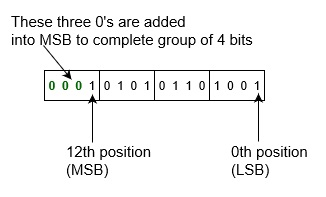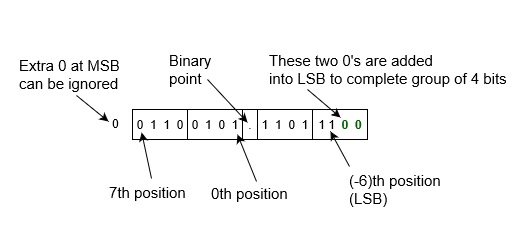# How to Convert Binary to Hexadecimal?

Binary is the simplest kind of number system that uses only two digits of 0 and 1 (i.e. value of base 2). Since digital electronics have only these two states (either 0 or 1), so binary number is most preferred in modern computer engineer, networking and communication specialists, and other professionals.

Whereas Hexadecimal number is one of the number systems which has value is 16 and it has only 16 symbols − 0, 1, 2, 3, 4, 5, 6, 7, 8, 9 and A, B, C, D, E, F. Where A, B, C, D, E and F are single bit representations of decimal value 10, 11, 12, 13, 14 and 15 respectively.

## Conversion from Binary to Hexadecimal number system

Hexadecimal number system provides convenient way of converting large binary numbers into more compact and smaller groups. There are various ways to convert a binary number into hexadecimal number. You can convert using direct methods or indirect methods. First, you need to convert a binary into other base system (e.g., into decimal, or into octal). Then you need to convert it hexadecimal number.

Most Significant Bit (MSB)Hexa PointLeast Significant Bit (LSB)
16216116016-116-216-3
2561611/161/2561/4096

Since number numbers are type of positional number system. That means weight of the positions from right to left are as 160, 161, 162, 163and so on. for the integer part and weight of the positions from left to right are as 16-1, 16-2, 16-3and so on. for the fractional part.

Example − Convert binary number 1101010 into hexadecimal number.

First convert this into decimal number:
= (1101010)2
= 1x26+1x25+0x24+1x23+0x22+1x21+0x20
= 64+32+0+8+0+2+0
= (106)10
Then, convert it into hexadecimal number
= (106)10
= 6x161+10x160
= (6A)16 which is answer.

However, there is also a direct method to convert a binary number into hexadecimal number − grouping which is explained as following below.

## Using Grouping

Since, there are only 16 digits (from 0 to 7 and A to F) in hexadecimal number system, so we can represent any digit of hexadecimal number system using only 4 bit as following below.

 Hexa 0 1 2 3 4 5 6 7 Binary 0000 0001 0010 0011 0100 0101 0110 0111

 Hexa 8 9 A B C D E F Binary 1000 1001 1010 1011 1100 1101 1110 1111

So, if you make each group of 4 bit of binary input number, then replace each group of binary number from its equivalent hexadecimal digits. That will be hexadecimal number of given number. Note that you can add any number of 0’s in leftmost bit (or in most significant bit) for integer part and add any number of 0’s in rightmost bit (or in least significant bit) for fraction part for completing the group of 4 bit, this does not change value of input binary number.

So, these are following steps to convert a binary number into hexadecimal number.

• Take binary number

• Divide the binary digits into groups of four (starting from right) for integer part and start from left for fraction part.

• Convert each group of four binary digits to one hexadecimal digit.

This is simple algorithm where you have to grouped binary number and replace their equivalent hexadecimal digit.

Example-1 − Convert binary number 1010101101001 into hexadecimal number. Since there is no binary point here and no fractional part. So,= (1010101101001)2
= (1 0101 0110 1001)2
= (0001 0101 0110 1001)2
= (1 5 6 9)16
= (1569)16

Example-2 − Convert binary number 001100101.110111 into hexadecimal number. Since there is binary point here and fractional part. So,= (001100101.110111)2
= (65.DC)16